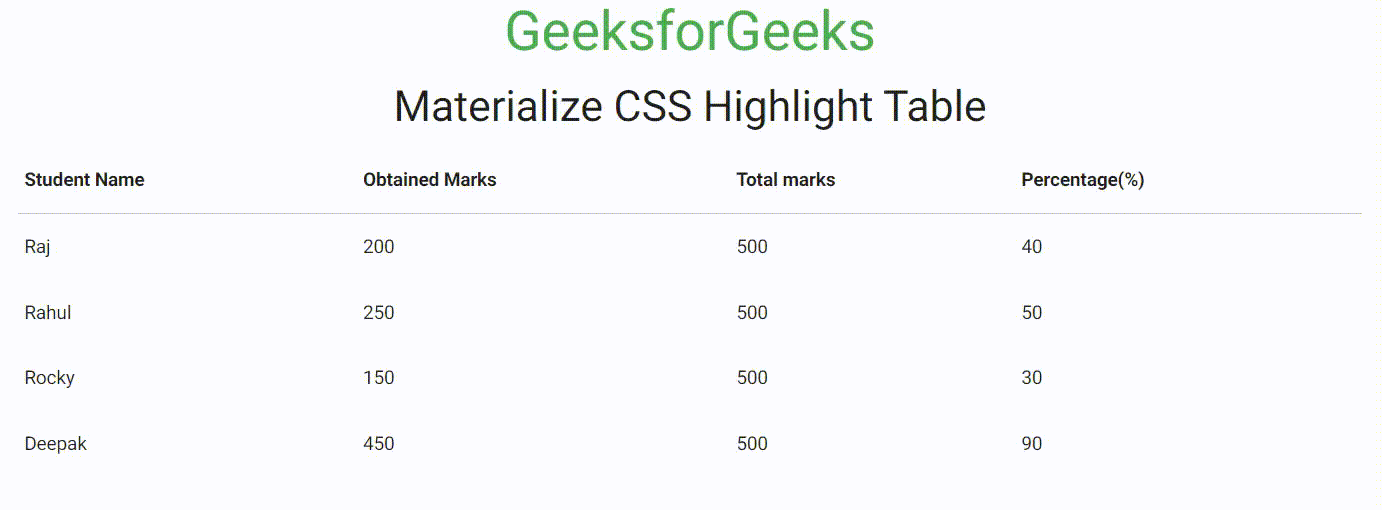GFG App
Open AppBrowser
Continue

# Materialize CSS Highlight Table

Materialize CSS is a design language that combines the classic principles of successful design along with innovation and technology. It is created and designed by Google. It is very easy to use to make our webpage dynamic and responsive.

Materialize CSS Table is used to store the data in the rows and columns in a well-structured manner. Materialize CSS Highlight Table is used to highlight the table rows when hovered with the help of the highlight class. In this article, we will discuss Highlight Table in Materialize CSS.

Materialize CSS Highlight Table Class:

• highlight: This class is used to highlight the rows when hovered over the table rows.

Syntax:

```<table class="highlight">
...
</table>```

Example: The following code demonstrates the Materialize CSS Highlight Table.

## HTML

 ` ` `<``html``> ` ` `  `<``head``> ` `    ``` `    ``<``link` `rel``=``"stylesheet"` `href``= ` `"https://cdnjs.cloudflare.com/ajax/libs/materialize/0.97.5/css/materialize.min.css"``> ` ` `  `    ``` `    ``<``meta` `name``=``"viewport"` `content``= ` `        ``"width=device-width, initial-scale=1.0"` `/> ` ` ` ` `  `<``body``> ` `    ``<``div` `class``=``"container center-align"``> ` `        ``<``h3` `class``=``"green-text"``> GeeksforGeeks ` `        ``<``h4``> Materialize CSS Highlight Table ` ` `  `        ``<``table` `class``=``"highlight"``> ` `            ``<``thead``> ` `                ``<``tr``> ` `                    ``<``th``> Student Name ` `                    ``<``th``> Obtained Marks ` `                    ``<``th``> Total marks ` `                    ``<``th``> Percentage(%) ` `                `` ` `            `` ` ` `  `            ``<``tbody``> ` `                ``<``tr``> ` `                    ``<``td``> Raj ` `                    ``<``td``> 200 ` `                    ``<``td``> 500 ` `                    ``<``td``> 40 ` `                `` ` `                ``<``tr``> ` `                    ``<``td``> Rahul ` `                    ``<``td``> 250 ` `                    ``<``td``> 500 ` `                    ``<``td``> 50 ` `                `` ` `                ``<``tr``> ` `                    ``<``td``> Rocky ` `                    ``<``td``> 150 ` `                    ``<``td``> 500 ` `                    ``<``td``> 30 ` `                `` ` `                ``<``tr``> ` `                    ``<``td``> Deepak ` `                    ``<``td``> 450 ` `                    ``<``td``> 500 ` `                    ``<``td``> 90 ` `                `` ` `            `` ` `        `` ` `    `` ` ` ` ` `  ``

Output:Materialize CSS Highlight Table

My Personal Notes arrow_drop_up